## Monday, September 27, 2010

### Fortune-telling

One program that some new users of Unix-like systems are introduced to when learning the command-line is fortune. The purpose of the program is simple: to display a random message from a database of quotations.

Note: fortune is available on the Mac OS X using MacPorts (`port install fortune`), Ubuntu (`apt-get install fortune-mod`), and Fedora (`yum install fortune-mod`).

A demonstration of running it:

```\$ fortune
The human race has one really effective weapon, and that is laughter.
-- Mark Twain```

I thought it would be fun to get this to work in Factor. We're going to do it two different ways. The first way is to use the io.launcher vocabulary for launching processes and printing the output directly to the listener.

```( scratchpad ) "/opt/local/bin/fortune" utf8
It'll be just like Beggars Canyon back home.
-- Luke Skywalker```

Another way is to parse the fortune files directly, and then choose a random quotation to print (i.e., implementing the fortune logic inside of Factor). First, we will need to list the vocabularies we will be using and define a "fortune" namespace:

```USING: io io.encodings.ascii io.files kernel make memoize
random sequences splitting strings ;

IN: fortune```

The fortune program uses data files which are stored in a "cookie-jar format". From faqs.org:

It is appropriate for records that are just bags of unstructured text. It simply uses newline followed by %% (or sometimes newline followed by %) as a record separator.

Parsing a fortune data file (given a string containing the contents in cookie-jar format) is pretty straightforward. We are using slices which allow us to keep pointers to sections of text within the data file.

```: parse-fortune ( str -- seq )
[
[ "%\n" split1-slice dup ]
[ swap , ] while drop ,
] { } make ;

: load-fortunes ( path -- seq )
ascii file-contents parse-fortune ;```

The fortune data files are installed in different locations on different systems, so we can define a search path which includes some of the locations that are often used. We can then use this list to load the fortunes from the first data file that exists (and memoize the results for performance):

```CONSTANT: FORTUNES {
"/usr/games/fortune/fortunes"
"/usr/share/games/fortune/fortunes"
"/usr/local/share/games/fortune/fortunes"
"/opt/local/share/games/fortune/fortunes"
}

MEMO: default-fortunes ( -- seq )
FORTUNES [ exists? ] find nip load-fortunes ;```

Getting a fortune is then just a matter of choosing a random quotation and printing it out:

```: fortune ( -- )
default-fortunes random >string print ;```

If you want, you could define a MAIN: entry point and use the deploy tool to make this into a deployed binary.

The source code for this is available on my Github.

Update: Doug Coleman suggested a one-liner that can produce the same result:

```"/opt/local/share/games/fortune/fortunes" ascii file-lines
{ "%" } split random [ print ] each```

## Wednesday, September 8, 2010

### Visual REPL

Many of the recently successful programming languages have a REPL ("read-eval-print-loop") for experimenting or iterative programming. Mostly these seem to be for dynamic languages, although there is even a C REPL! Almost invariably, these are text-based (with notable exceptions for math systems like Mathematica).

I thought it would be fun to highlight some of the visual aspects of Factor's REPL (called the "listener"). For the examples below, it would be helpful to use these vocabularies first:

`( scratchpad ) USING: colors http.client images.http io.styles ;`

## Styled Text

Print styled text directly to the listener's output. You can change font sizes, foreground and background colors, font family, font style, and other aspects of the text: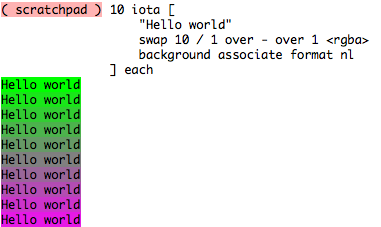## Tab Completion

You can get word completion as you write code (by pressing `TAB`) in the listener: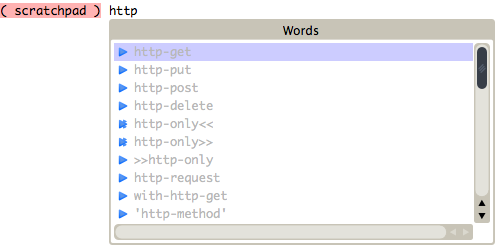## Word Definitions

You can print the definition of any word using `see`. In addition, you can click on most parts of the output to link to vocabulary pages, word definitions, etc.: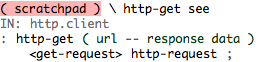Use apropos to search for words, vocabularies, and help articles. It uses some fuzzy completion algorithms to be more helpful for typos or approximations.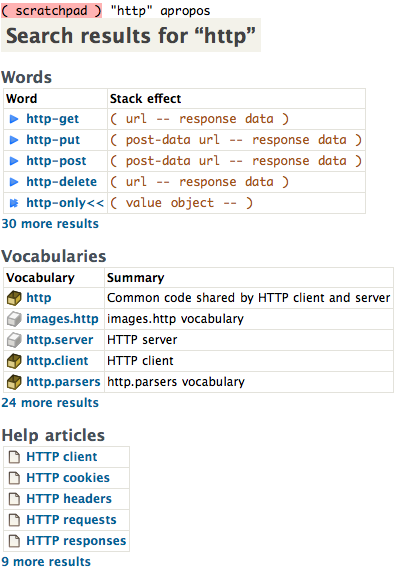## Viewing Images

Use the handy `images.viewer` vocabulary (written by Doug Coleman) to load and display images directly in the listener: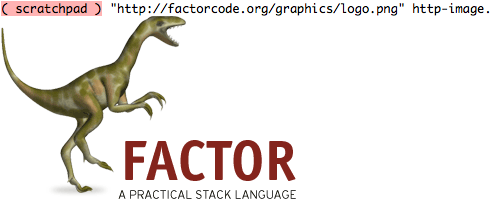## Monday, September 6, 2010

### Approximating Rationals

A few months ago, there was an article about approximating numbers with fractions using the `approxRational` function in Haskell. The documentation for the function states:

`approxRational`, applied to two real fractional numbers `x` and `epsilon`, returns the simplest rational number within `epsilon` of `x`. A rational number `y` is said to be simpler than another `y'` if

1. `abs (numerator y) <= abs (numerator y')`, and
2. `denominator y <= denominator y'`.

Any real interval contains a unique simplest rational; in particular, note that `0/1` is the simplest rational of all.

The source code for `approxRational` is:

```approxRational          :: (RealFrac a) => a -> a -> Rational
approxRational rat eps  =  simplest (rat-eps) (rat+eps)
where simplest x y | y < x      =  simplest y x
| x == y     =  xr
| x > 0      =  simplest' n d n' d'
| y < 0      =  - simplest' (-n') d' (-n) d
| otherwise  =  0 :% 1
where xr       = toRational x
n        = numerator xr
d        = denominator xr
nd'      = toRational y
n'       = numerator nd'
d'       = denominator nd'

simplest' n d n' d'       -- assumes 0 < n%d < n'%d'
| r == 0     =  q :% 1
| q /= q'    =  (q+1) :% 1
| otherwise  =  (q*n''+d'') :% n''
where (q,r)    =  quotRem n d
(q',r')  =  quotRem n' d'
nd''     =  simplest' d' r' d r
n''      =  numerator nd''
d''      =  denominator nd''
```

I couldn't find an equivalent algorithm in Factor, so I decided to translate it (using lexical variables to make the comparison between the languages as direct as possible).

```USING: combinators kernel locals math math.functions ;

:: (simplest) ( n d n' d' -- val ) ! assumes 0 < n/d < n'/d'
n  d  /mod :> ( q  r  )
n' d' /mod :> ( q' r' )
{
{ [ r zero? ] [ q ] }
{ [ q q' = not ] [ q 1 + ] }
[
d' r' d r (simplest) >fraction :> ( n'' d'' )
q n'' * d'' + n'' /
]
} cond ;

:: simplest ( x y -- val )
{
{ [ x y > ] [ y x simplest ] }
{ [ x y = ] [ x ] }
{ [ x 0 > ] [ x y [ >fraction ] bi@ (simplest) ] }
{ [ y 0 < ] [ y x [ neg >fraction ] bi@ (simplest) neg ] }
[ 0 ]
} cond ;

: approximate ( x epsilon -- y )
[ - ] [ + ] 2bi simplest ;
```

We can experiment with the function:

```( scratchpad ) 33/100 1/10 approximate .
1/3

( scratchpad ) 1/3 1/10 approximate .
1/3
```

We can use the code I posted a few days ago to convert floating-point numbers to fractions. That will be useful for running the experiment from the original blog post (to approximate `pi` with varying degrees of accuracy):

```( scratchpad ) USING: math.constants math.statistics math.vectors ;
( scratchpad ) 10000 [1,b] [ 0.99 swap ^ float>ratio ] map
( scratchpad ) pi float>ratio '[ _ swap approximate ] map
( scratchpad ) sorted-histogram reverse .
{
{ 3+39854788871587/281474976710656 3447 }
{ 3+16/113 572 }
{ 3+464086704149/3277618523158 433 }
{ 3+1/7 297 }
{ 3+244252/1725033 288 }
{ 3+1470786781/10387451211 248 }
{ 3 194 }
{ 3+11080585/78256779 186 }
{ 3+56667685227/400216280932 176 }
{ 3+449714866/3176117225 172 }
}
```

Looking at the top 10 most common approximations, you can see the first (and most frequent) result is the exact fractional value of the constant `pi`, the fourth result is the common "grade school" estimate `22/7`, and the second result is the "best" simple estimate `355/113`.

Note: the output is slightly different than the Haskell program. Factor considers several of these rational numbers to be equal when converted to floating-point:

• `3+39854788871587/281474976710656`
• `3+464086704149/3277618523158`
• `3+1470786781/10387451211`
• `3+11080585/78256779`
• `3+56667685227/400216280932`

The code (and some tests) for this is on my Github.

## Saturday, September 4, 2010

### Internet Checksum

I needed to calculate the "internet checksum" for a small project I've been working on. While Factor includes several checksum algorithms, it didn't have support (until recently) for the "internet checksum".

The "internet checksum" is a 16-bit checksum value specified in RFC 1071 and used in many places within standard network protocols (such as the IP header). The RFC includes this description of the algorithm:

Adjacent octets to be checksummed are paired to form 16-bit integers, and the 1's complement sum of these 16-bit integers is formed.

Some C code is provided in the RFC as an example of the algorithm for performing this computation (including comments):

```/* Compute Internet Checksum for "count" bytes
*/
register long sum = 0;

while( count > 1 )  {
/*  This is the inner loop */
sum += * (unsigned short) addr++;
count -= 2;
}

/*  Add left-over byte, if any */
if( count > 0 )
sum += * (unsigned char *) addr;

/*  Fold 32-bit sum to 16 bits */
while (sum>>16)
sum = (sum & 0xffff) + (sum >> 16);

checksum = ~sum;```

In Factor, we can use some high-level concepts like grouping to walk through the bytes two at a time. In fact, the entire implementation is only a few lines and pretty readable:

```: internet-checksum ( bytes -- value )
2 <sliced-groups> [ le> ] map-sum
[ -16 shift ] [ HEX: ffff bitand ] bi +
[ -16 shift ] keep + bitnot 2 >le ;```

This is available as a `checksum` instance in checksums.internet.

## Thursday, September 2, 2010

### What can you get from 1, 2, 3, 4, +, -, *, /, and ^?

Recently, a Haskell program was posted that computed all possible combinations of the numbers "1, 2, 3, and 4" and the operators "+, -, *, /, and ^" (allowing the operators to be repeated, but not the numbers). It's a fun little problem, and I thought it might be a good example for iterative development in Factor using the listener.

First, some vocabularies that we'll be using.

```( scratchpad ) USING: arrays continuations kernel math
math.combinatorics math.statistics sequences ;```

We can calculate all-permutations of the numbers (for a total of 4!, or 24):

`( scratchpad ) { 1 2 3 4 } all-permutations`

And, similarly, we can find all possible selections of three operations (for a total of 53, or 125):

`( scratchpad ) { + - * / ^ } 3 selections`

Now, we can compute the cartesian-product to produce all possible pairings of the two sequences (for a total of 24 × 125, or 3000):

`( scratchpad ) cartesian-product concat`

You can inspect the list by clicking on it in the listener, printing it out (e.g., "`dup .`", or showing what the first result looks like:

```( scratchpad ) dup first .
{ { 1 2 3 4 } { + + + } }```

Use concat-as to make all the entries quotations (so they can be called).

`( scratchpad ) [ [ ] concat-as ] map`

We can then try calling the first element to make sure it produces the right result:

```( scratchpad ) dup first dup . call .
[ 1 2 3 4 + + + ]
10```

Let's call each quotation, creating an association list where the key is the quotation and the value is the result:

```( scratchpad ) [ dup call 2array ] map
Division by zero
x 2```

Whoops, some of the formulas produce division-by-zero errors. We can use continuations to recover (storing a `f` result when there is an error) and continue:

`( scratchpad ) [ [ dup call ] [ drop f ] recover 2array ] map`

Each element of the resulting sequence is a pairing of a quotation and a result:

```( scratchpad ) dup first .
{ [ 1 2 3 4 + + + ] 10 }```

We can see how many unique results (including `f`) are found:

```( scratchpad ) dup values unique assoc-size .
430```

You could calculate the 10 most common results using sorted-histogram. It turns out "1" is the most common result:

```( scratchpad ) dup values sorted-histogram reverse 10 head .
{
{ 1 200 }
{ 4 116 }
{ 2 116 }
{ 3 96 }
{ 6 82 }
{ 9 65 }
{ 5 60 }
{ -2 57 }
{ 24 56 }
{ 1+1/2 53 }
}```

Some other things you might try:

• Count how many "divide-by-zero" errors are produced, perhaps using assoc-filter to examine the "bad" formulas.
• Create an association between each unique value and the list of all quotations that produced it.
• Print each quotation and result out, sorted by numerical result.
• Define a word that, given a sequence of numbers and a sequence of operations, produces all the result pairings (using call( to make it compile properly).
• Find the most positive and most negative result, and output the quotations that produced them.

Update: it was pointed out by Kevin Reid (the author of the Haskell version) that I'm missing left-associative operators. I think the only modification that is required is to add "swapped" versions of the operators to the possible choices:

```( scratchpad ) USE: quotations

( scratchpad ) { + - * / ^ } [ 1quotation ] map

( scratchpad ) dup [ [ swap ] prepend ] map append dup .
{
[ + ]
[ - ]
[ * ]
[ / ]
[ ^ ]
[ swap + ]
[ swap - ]
[ swap * ]
[ swap / ]
[ swap ^ ]
}

( scratchpad ) 3 selections [ concat ] map```

This produces 103, or 1,000, possible operations. When combined with the original 24 permutations of `{ 1 2 3 4 }`, that makes 24,000 possible formulas. Running through the logic above makes 677 unique results. Still not sure why this is close, but doesn't quite match, the original results in Haskell.

Update 2: it was pointed out by joop that addition and multiplication don't need "swapped" versions.

Update 3: it was pointed out by Scaevolus that I am missing `(4 ^ 4) ^ (4 ^ 4)`. In Factor, that could be represented by `[ 4 4 ^ 4 4 ^ ^ ]` or `[ 4 4 4 4 ^ [ ^ ] dip ^ ]`. One fix would be to include the "dipped" operators in the first two positions:

```( scratchpad ) {
[ + ] [ - ] [ * ] [ / ] [ ^ ]
[ swap - ] [ swap / ] [ swap ^ ]
[ [ - ] dip ] [ [ swap - ] dip ]
[ [ / ] dip ] [ [ swap / ] dip ]
[ [ ^ ] dip ] [ [ swap ^ ] dip ]
} 2 selections